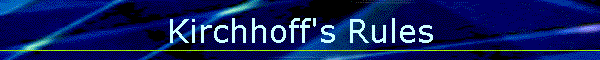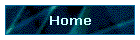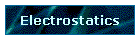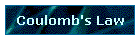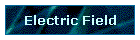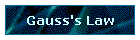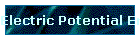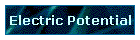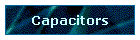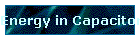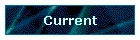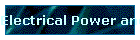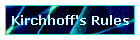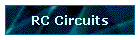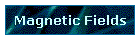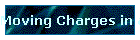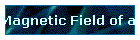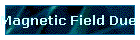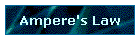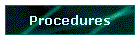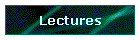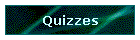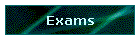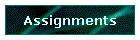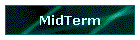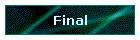Phys 4, Section 2                                                    Feb 6, 2002

# Kirchhoff's Rules

The sum of the currents into any circuit junction is zero.
The sum of the potential differences around any closed circuit loop is zero.

Notes:

There is more than one way to break up a circuit into loops.
Every component must be in at least one loop.
Current flowing through a resistor causes a voltage drop.
Ideal batteries have no internal resistance.

Procedure for applying Kirchhoff's Rules:

Break the circuit up into loops.
Choose the (arbitrary) direction of current flow around each loop.
Use Kirchhoff's second rule to get an equation for each loop.
Solve the set of simultaneous equations.
There can be as many unknowns as there are equations.